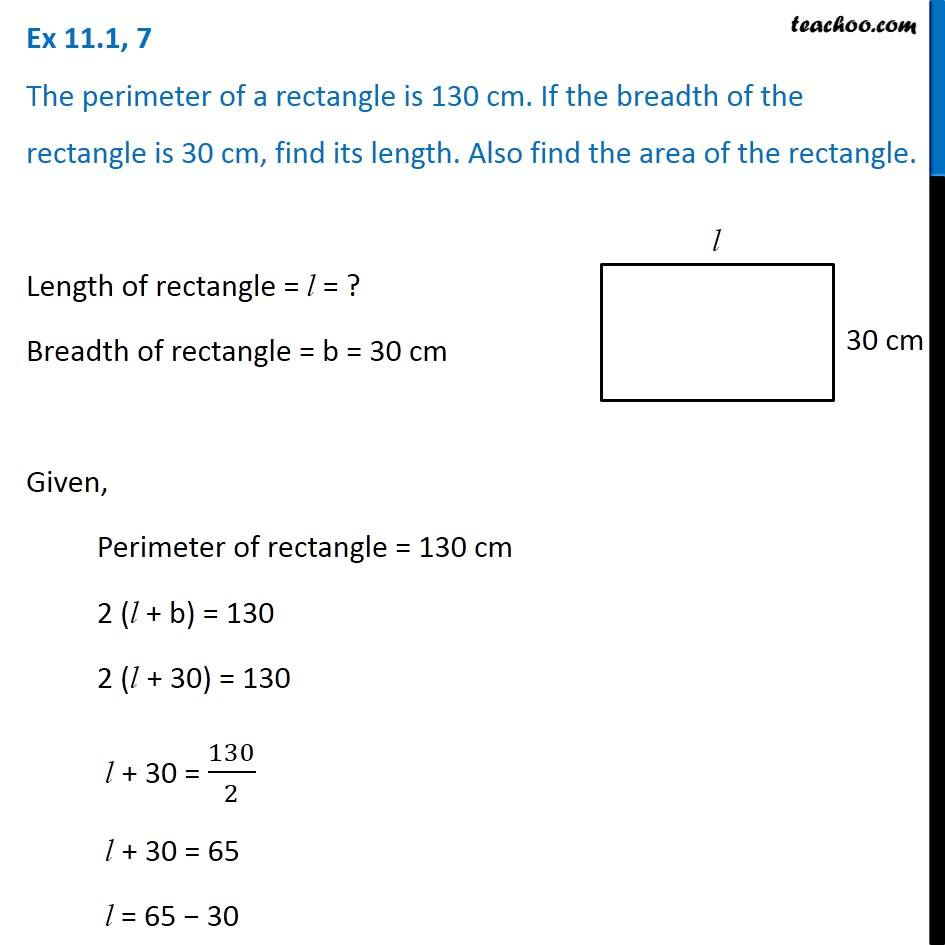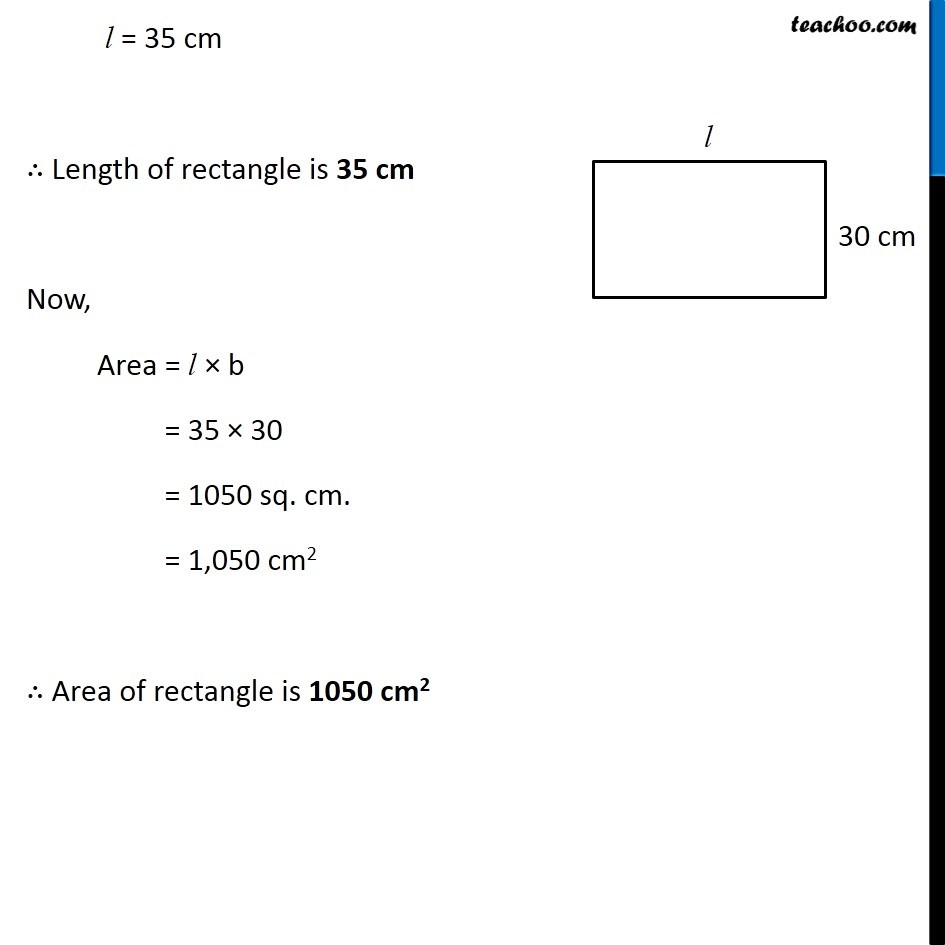Area of Square and Rectangles - Worksheet

Chapter 9 Class 7 Perimeter and Area
Serial order wiseLearn in your speed, with individual attention - Teachoo Maths 1-on-1 Class

### Transcript

Question 7 The perimeter of a rectangle is 130 cm. If the breadth of the rectangle is 30 cm, find its length. Also find the area of the rectangle. Length of rectangle = l = ? Breadth of rectangle = b = 30 cm Given, Perimeter of rectangle = 130 cm 2 (l + b) = 130 2 (l + 30) = 130 l + 30 = 130/2 l + 30 = 65 l = 65 − 30 l = 35 cm ∴ Length of rectangle is 35 cm Now, Area = l × b = 35 × 30 = 1050 sq. cm. = 1,050 cm2 ∴ Area of rectangle is 1050 cm2